4/12/2021

## Gameplay Sketching

Sketching Levels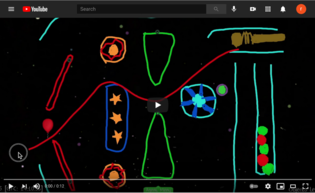Physics Playground Sketching

Players draw objects (ramps, pendulums, levers, springboards) to get ball to balloon.

## Gameplay Manipulation

Manipulation Levels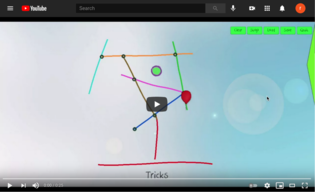Physics Playground Manipulation

Players manipulate physics parameters (gravity, ball mass, air resistance, bounciness) to get ball to balloon.

## Four Process Architecture

• Presentation (Game Engine) – Presents game level captures work product (collection of events).

• Evidence Identification (Server) – Processes events for a game level to create observable evidence sets for the game levels.

• Evidence Accumulation (Server) – Uses Bayesian networks to estimate physics proficiencies from game levels.

• Activity Selection (Game Engine) – Picks next game level based on current ability estimates.

Note: Events and evidence sets are stored in a database, so scoring can easily be rerun by marking evidence sets as “unprocessed” and reprocessing.

## Physics Playground Model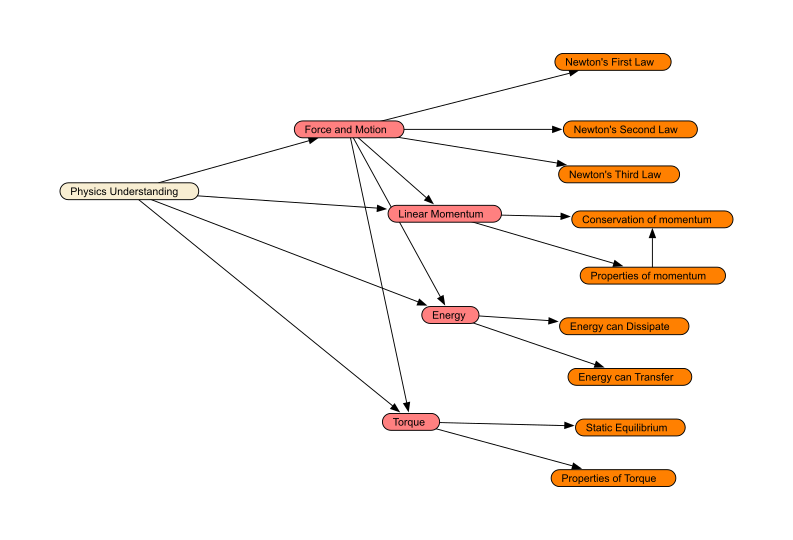Proficiency Model

• 4 high-level proficiencies (salmon)
• 9 low-level proficiencies (orange).

Each nodes has states High, Medium and Low.

## Physics Playground Q-Matrix

There is a collection of evidence models: one for each game level.

These are represented through an augmented Q-matrix

online sheet

Peanut package has code for building Bayes nets from spreadsheet.

## Physics Playground Scores

• Margin – Probabilities for High, Medium and Low (vector valued).

• Mode – One of High, Medium or Low with highest probability.

• EAP (Expected A Posteriori) – Assign the value +1 to High, 0 to Medium and -1 to Low and then calculate the expected value. This can be also expressed as $$\Pr(High) -\Pr(Low)$$. The score runs from +1 (high) to -1 (low). (In the implementation, .97 and -.97 was used, so the actual scores do not every quite reach +1 or -1).

EABN Engine can spit out each of these score types for each node.

## Split Halfs

• “Items” are game levels: need to leave game levels intact.

• Pair game levels on:

• Primary and Secondary Skills
• Difficulty
• Other features
• Randomly assign one level of each pair to Form A and Form B.

## Rescoring Logic

• Mark observable sets from Form A levels as unscored. Rescore to get Form A scores.

• Mark observable sets from Form B levels as unscored. Rescore to get Form B scores.

## Reliabilities: EAP correlations

EAP scores are continuous, so can use ordinary correlations.

Optionally apply Spearman-Brown corrections

Correlations between Form A and B sub-forms.
Measure Reliability
Physics 0.229
Force and Motion 0.135
Linear Momentum 0.080
Energy 0.456
Torque 0.139

## Scatterplot (Higher Reliability)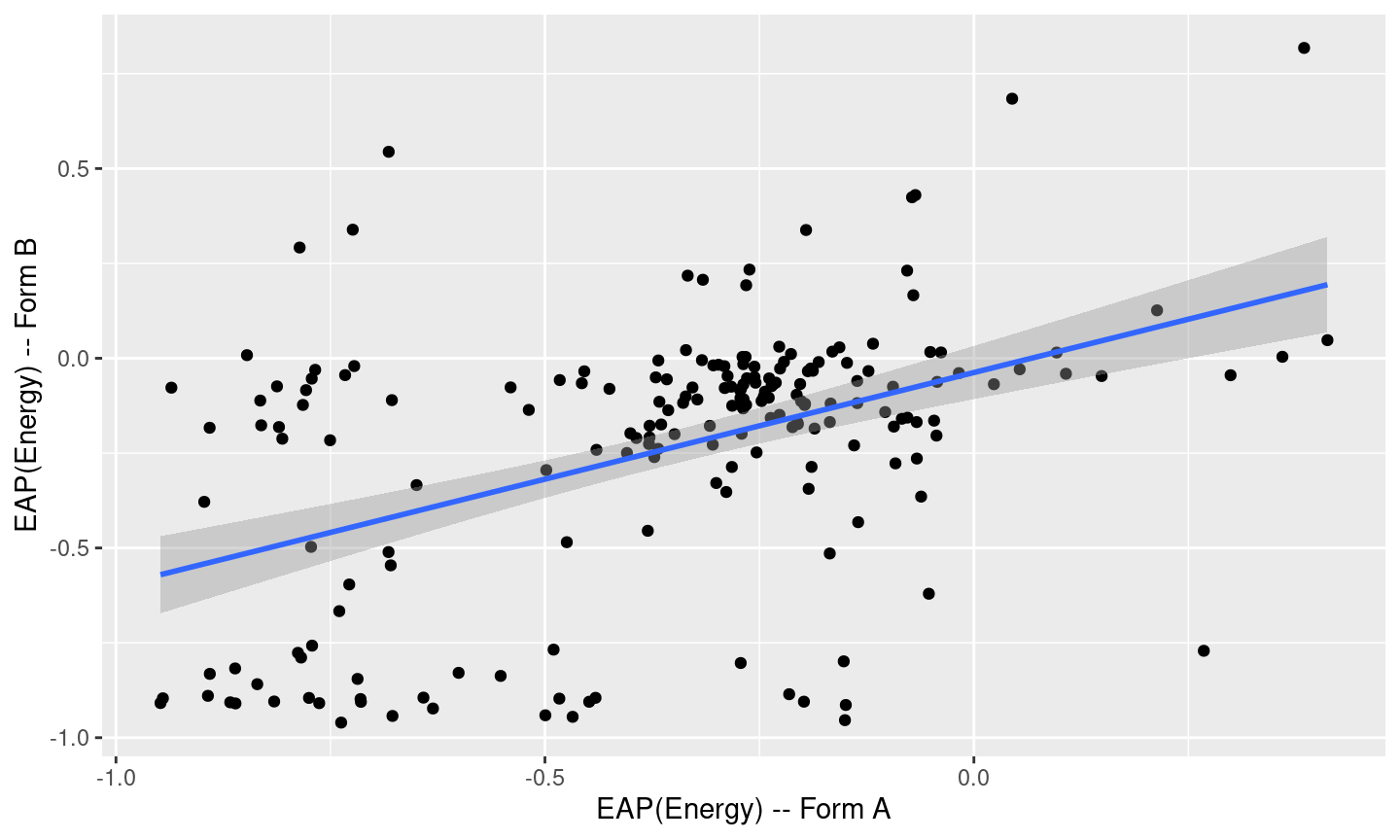Energy EAP score consistency

## Scatterplot (Lower Reliability)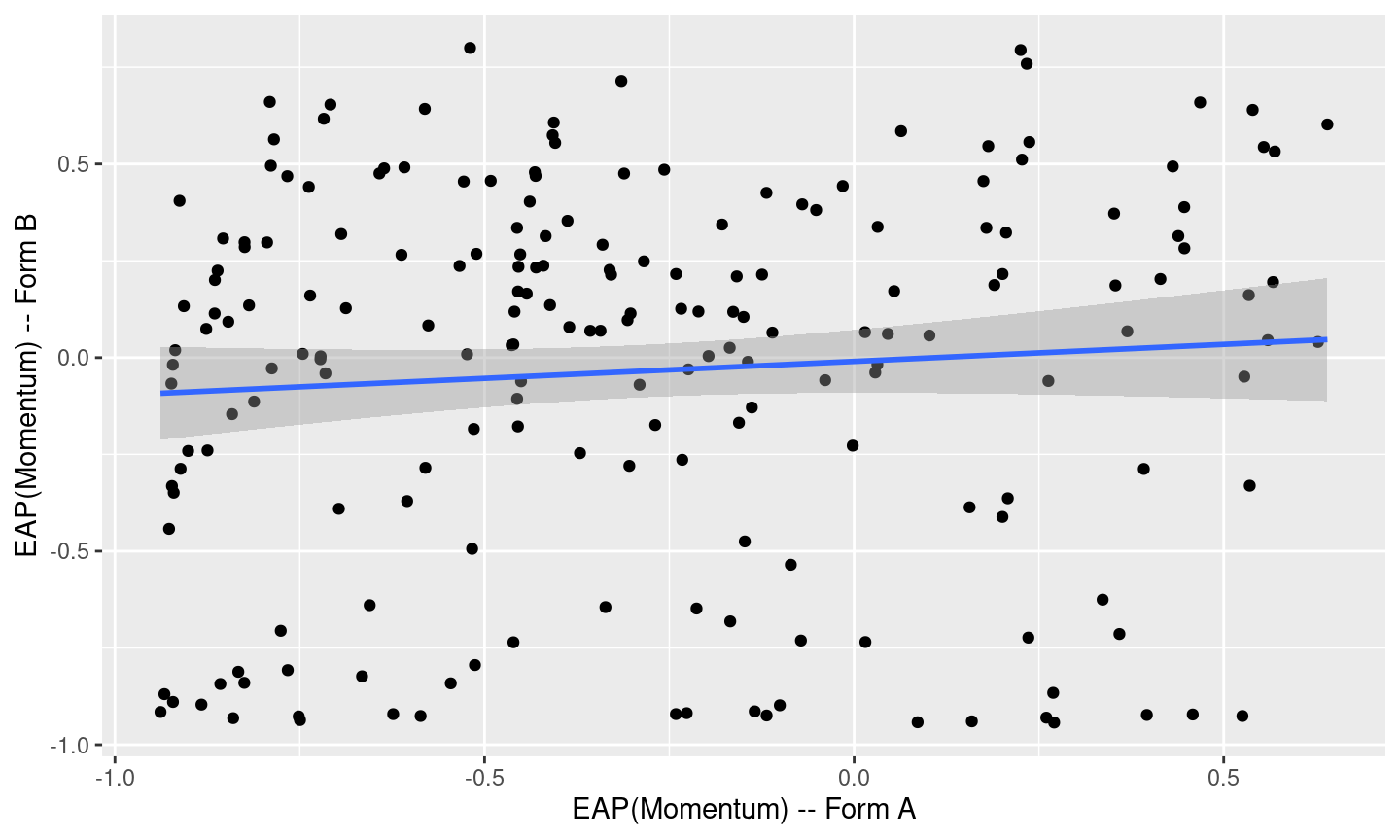Linear Momentum EAP score consistency

## Reliabilities: Modal Kappas

modalTab <- table(statAB$Physics_ModeA,statAB$Physics_ModeB)
Agreement of Physics Modal Scores.
L M
L 53 89
M 17 40
## Call: cohen.kappa1(x = x, w = w, n.obs = n.obs, alpha = alpha, levels = levels)
##
## Cohen Kappa and Weighted Kappa correlation coefficients and confidence boundaries
##                  lower estimate upper
## unweighted kappa -0.05    0.054  0.16
## weighted kappa   -0.05    0.054  0.16
##
##  Number of subjects = 199

The cohen.kappa function is from the psych package.

## Expected Kappas (1)

Get the “expected agreement” table by multiplying the margin scores together.

statAB[1,paste("Physics_Margin.",c("High","Medium","Low"),"A",sep="")]
##   Physics_Margin.HighA Physics_Margin.MediumA Physics_Margin.LowA
## 1               0.0323                 0.6432              0.3244
statAB[1,paste("Physics_Margin.",c("High","Medium","Low"),"B",sep="")]
##   Physics_Margin.HighB Physics_Margin.MediumB Physics_Margin.LowB
## 1                5e-04                 0.0941              0.9055

## Expected Kappas (2)

outer(
as.numeric(statAB[1,paste("Physics_Margin.",c("High","Medium","Low"),"A",sep="")]),
as.numeric(statAB[1,paste("Physics_Margin.",c("High","Medium","Low"),"B",sep="")])
)
##           [,1]       [,2]       [,3]
## [1,] 1.615e-05 0.00303943 0.02924765
## [2,] 3.216e-04 0.06052512 0.58241760
## [3,] 1.622e-04 0.03052604 0.29374420

## Expected Kappas (3)

Now sum accros students:

states <- c("High","Medium","Low")
expTab <- outer(
as.numeric(statAB[1,paste("Physics_Margin.",states,"A",sep="")]),
as.numeric(statAB[1,paste("Physics_Margin.",states,"B",sep="")])
)
for (irow in 2:(nrow(statAB))) {
itab <- outer(
as.numeric(statAB[irow,paste("Physics_Margin.",states,"A",sep="")]),
as.numeric(statAB[irow,paste("Physics_Margin.",states,"B",sep="")])
)
if (all(!is.na(itab)))
expTab <- expTab + itab
}
rownames(expTab) <- states
colnames(expTab) <- states

## Expected Kappas (4)

Agreement of Physics Marginal Scores.
High Medium Low
High 0.111 1.482 1.061
Medium 2.837 42.651 29.662
Low 3.485 62.450 55.261
## Call: cohen.kappa1(x = x, w = w, n.obs = n.obs, alpha = alpha, levels = levels)
##
## Cohen Kappa and Weighted Kappa correlation coefficients and confidence boundaries
##                   lower estimate upper
## unweighted kappa -0.073    0.050  0.17
## weighted kappa   -0.357    0.057  0.47
##
##  Number of subjects = 199

## Correlations with external measures

The Fall 2019 Field trial included a pret-test and post-test.

Items were coded to subscales matching the 4 high-level (salmon) nodes.

Correlation of Physics EAP score with whole pretest and posttest.
preScore postScore Physics_EAP
preScore 1.000 0.698 0.226
postScore 0.698 1.000 0.182
Physics_EAP 0.226 0.182 1.000

## Subscales

As the subscale measures are short, sum the pre -test and post-test scores

Reliability (sub-form correlations) and Valitity (corrlation with pretest + posttest)
Measure Reliability Validity
Physics 0.229 0.220
Force and Motion 0.135 0.153
Linear Momentum 0.080 0.061
Energy 0.456 0.223
Torque 0.139 0.174

## Eta Statistic

Can use the $$\eta$$ or $$\eta^2$$ statistic to show how much of variability in score is explained by classification.

## AOV expect factors.
BSEAP$Physics_Mode <- factor(BSEAP$Physics_Mode)
## Pretest
preaov <- aov(preScore ~ Physics_Mode,data=BSEAP)
cat("Pretest eta:", round(
sqrt(eta_squared(preaov, partial=FALSE)\$Eta_Sq),
digits=2),"\n")
## Pretest eta: 0.17
## Posttest eta: 0.14

The eta_squared function is from the effectsize package.

## Discussion

These techniques work with any CDM which provides probability distributions over mastery states.

ECD models really help to produce matched forms for split-halfs.

These calculations can be used in formatory analysis.

Can do this with simulated data to examine form designs.